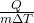# 10th Class Physical Science Chapter wise Important bit bank in English10th Class Physical Science (Physics) Chapter wise Important bit bank Bits in English. 10th Class Physical Science Chapter wise bit bank for Tenth Class (English Medium students) Heat, Chemical Reactions and Equations, Reflection of light on different surfaces, Acid Basis and Salts, Refraction of Light at Plane Surfaces, Refraction of Light at Curved Surfaces, Human Eye and Colourful world, Structure of Atom, Classification of Elements- The Periodic Table, Chemical Bonding, Electric Current, Electromagnetism, Principles of Metallurgy, Carbon and its Compounds and 10th Class Physical Science Important Bits for English Medium Students. This study material will useful a lot for all students. This study material contains chapter wise important bits for 10th class students as well as all competitive exams like POLYCET, APRJC GURUKULAM, DEECET. These questions are important objective multiple choice questions for 10th Class Social Studies Public Examinations in Telangana, Andhra Pradesh.

1. Heat

1. Heat is the form of __________ .

energy

2. “___________ and Heat” to describe the hot and cold.

Temperature

3. The state of ______________ denotes a state of a body where it neither receives nor gives out heat energy.

thermal equilibrium

4. ______ is the energy that flows from a hotter to a colder body.

Heat

5. The SI unit of heat is _________ and CGS unit is __________.

Joule (J), calorie (cal)

6. The amount of heat required to increase the temperature of 1gram of water by 10C is called ________.

calorie

7. 1Cal = _________ joules

4.186

8. The SI unit of temperature is ___________. It can also be expressed as degree _________.

Kelvin (K), Celsius (0C)

9. 00C = ________ K.

273

10. Temperature in Kelvin = 273+______________________.

Temperature in degree Celsius

11. Temperature measured in Kelvin scale is called ________________.

absolute temperature

12. The bodies possess ____________ when they are in motion.

kinetic energy

13. We know the rise in temperature depends on the nature of the ________.

material

14. The average kinetic energy of the molecules is directly proportional to the _________ temperature.

absolute

15. Temperature is a quantity that denotes which body is hotter and which is ________ .

colder

16. “Specific heat” of substance, S = __________. J/Kg-K.17. Specific heat gives us an idea of the degree of ‘_________’ of a substance to change its temperature.

reluctance

18. CGS unit of specific heat is cal/g-oC and SI unit of it is ________.

J / kg – K

19. 1 cal/g- oC = 1 kcal /kg-K= _________ J/kg-K

4.2 x 103

20. The oceans behave like heat “___________” for the earth.

store houses

21. Oceans can absorb large amounts of heat at the equator without much rise in
temperature due to ______________________.

high specific heat capacity of water

22. Water has greater __________ heat value.

specific

23. Net heat lost= Net heat gain. This is known as principle of method of _________.

mixtures

24. “The process of escaping of molecules from the surface of a liquid at any temperature is called __________”

evaporation

25. ________ is a surface phenomenon.

1.